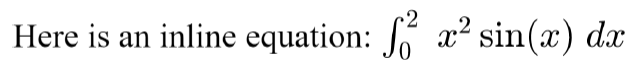Main Content

# Create an Inline Equation in a Report

This example shows how to insert an equation in a line of text in a report. For example:You indicate whether an equation is on a line by itself or in line with the adjacent text by setting the DisplayInline property of an equation reporter. If the DisplayInline property is set to false, the reporter adds an image of the formatted equation on a separate line of a report. If the DisplayInline property is set to true, you get the image of the formatted equation by calling the getImpl method and add the image to a paragraph in the report.

### Import API Packages

Import the DOM and Report API packages so that you do not have to use long, fully qualified class names.

import mlreportgen.report.* import mlreportgen.dom.* 

### Create Report

This example creates a single-file HTML report. To create a different type of report, change the output type to "html", "pdf", or "docx". Create a paragraph to contain the equation.

rpt = Report("myreport","html-file"); p = Paragraph("Here is an inline equation: "); p.FontSize = "14pt"; p.WhiteSpace = "preserve";

### Create an Equation Reporter for an Inline Equation

Create an Equation reporter. Specify that the image of the equation is in line with the adjacent text by setting the DisplayInline property to true.

eq = Equation("\int_{0}^{2} x^2\sin(x) dx"); eq.DisplayInline = true; eq.FontSize = 14;

### Add Image of Equation to Report

To get a snapshot image of the formatted equation, call the getImpl method. Align the baseline of the equation integrand with the baseline of the text by specifying an amount by which the image is lowered from the baseline of the text. Try different amounts until you are satisfied with the alignment. For HTML and PDF reports, you can specify the amount as a percentage of the line height. For Word reports, specify the amount as a number of units. See the Value property of the mlreportgen.dom.VerticalAlign class.

eqImg = getImpl(eq,rpt); if (rpt.Type == "html" || rpt.Type == "html-file" || rpt.Type == "pdf") eqImg.Style = {VerticalAlign("-30%")}; elseif(rpt.Type == "docx") eqImg.Style = {VerticalAlign("-5pt")}; end

Add the image to the paragraph. Add the paragraph to the report.

append(p,eqImg); add(rpt,p);

### Close and View Report

close(rpt); rptview(rpt);

## SupportGet trial now